•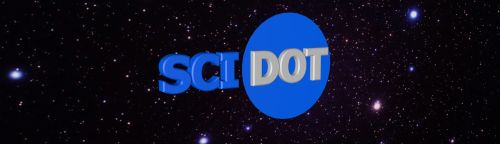•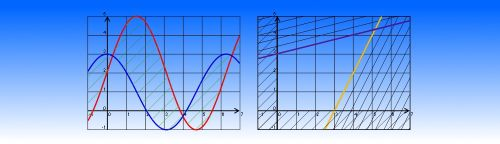••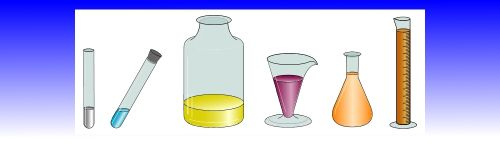•••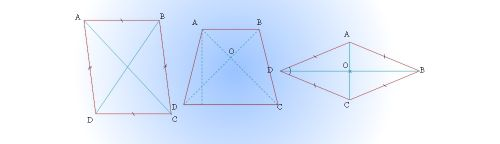•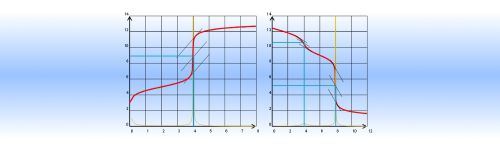## Features

MATHEMATICS

• EQUATION EDITOR
• Button to open Word Equation Editor
• Button to open a Simplified Equation Editor
• Button to toggle between Word Equation Editor and Fields Codes
• Button to display/hide Fields Code
• Mathematical formatting using either Word Equation Editor or Fields Codes (button and context menu)
• Insert an angle, a vector, a fraction, a square (or multiple) root, text bordered with a frame, an arc, a conjugate, an algebraic measurement, cross out a text, simplify a fraction, insert a multiplication arrow, insert superscript and subscript simultaneously
• Insert a matrix, calculator keys, delimitors (parenthesis, brackets, rounded brackets)
• Insert a sum, a product, an integral sum, an arrangement, a combinaison (2 forms), a primitive form, a limit, a derivative, an union, an intersection, an equivalent
• Insert set symbols R, N, Z, C, Q, F, D, +/-/* and most common symbols
• Insert greek letters and cursives caps.

• MATHEMATICAL OBJECTS
• draw additions, subtractions, multiplications, divisions as done with the pencil
• Compute the expression of a function from given points (line, parabola, cubic function)
• Insert or fill table of values for one or many functions
• Draw a table of variations of a function (defining the function expression or not)
• Draw a table of sign of one or many expressions
• Draw functions curves, conics, lines, vectors, etc...
• Statistics
• histograms, bar graph, pie chart, cumulative frequency
• compute tables of statistics values
• draw box plot : decils, min/max, Q1Q3
• plot X-Y with linear regression

• MATHEMATICAL TOOLS
• Mini scientific calculator (save expression, compose function, compute derivative)

• GEOMETRY
• Draw a point, a segment, a double-arrow, remarkable lines of a selected polygon,
• Draw a grid to draw the following elements: point, vector, line, function curves, conics, cloud of points
• Draw an angle of line, a triangle, a trapezoid, a parallelogram, a rhombus, a square, a rectangle, a regular polygone
• Draw a circle, an arc of circle, a pie-chart, simple statistical drawing (bar graph, pie chart, half-pie chart)
• Draw all kind of grids (small and large school paper, hexagonal, rectangle, triangular pattern), and networks of points
• Draw millimeter paper, semi-logarithmic and logarithmic paper, polar paper, axis and trigonometric circle
• Represent a fraction with a disc, a square, a rectangle
• Draw 3D objects (tetrahedron, cube, pavoid, pyramid, prism, cone, cylinder, sphere, intersection between the earth and a plane, the earth globe)
• Draw Perspectives (cube, pavoid, cylinder, prism, pyramid)
• Draw Folding (tetrahedron, cube, pavoid, pyramid, prism, cone, cylinder)
• Draw usual instruments: rule, compass, set square, protractor, pen (fits on the selected line segment)
• Measure the length, the perimeter, the angle and the area of a polygon (triangle, parallelogram, etc..)
• Apply transformations of the plane to drawing objects: central symmetry, axial symmetry, rotation, translation, dilation

• PHYSICS
• Creates electricity & electronic drawings that fit on a grid
• More drawings in electromagnism, semi-conductors, pneumatics
• Creates mechanical, optical, acoustical drawings
• Oscilloscope screen editor: 4 inputs with pre-traced curves and function graphing
• Optical bank: draw rays through one or two converging or diverging lenses ; common optical objects (camera, atronomical lunette, eye...)
• Spectrum: draw spectrum of emitting and absorbing rays of chemical elements, draw the light spectrum

• CHEMISTRY
• Quick insertion of ions, atoms, Ox/Red, and Acido-basic couples (with constant)
• 2D Chemistry glassware and lab-equipment
• 3D Chemistry glassware and lab-equipment: usual titration and equipments
• Pictograms, symbols of danger, and new symbols of danger
• Compute molar mass, print chemical symbols
• Draw evolution table of a chemical reaction
• Graphs titration curves (pH-meter and conductimeter method), predominance diagrams and axes
• Chemical reaction arrows
• Chemical formula editor (molecular and structural)

• PHYSICS AND CHEMISTRY TOOLS
• graph up to 4 curve
• scale different along x-axis and y-axis
• scientific formatting
• graph cartesian and parametric curves
• draw tangents
• hash area defined by one or two functions
• conics
• Build your own library of drawings
• Specific symbols for physics and chemistry